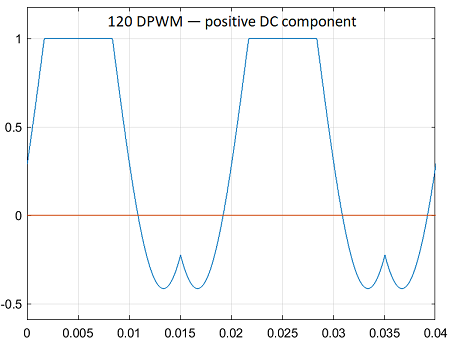PWM Reference Generator

Generate modulated signals from phase voltages

• Library:
• Motor Control Blockset / Controls / Math Transforms

•Description

The PWM Reference Generator block generates modulated voltage signals from the stator phase or reference voltages.

The block accepts either the phase voltages (Vabc) or the stator reference voltages (Vαβ) described by the α-β voltage components.

Use this block to perform sinusoidal PWM (SPWM) and space vector modulation (SVM) along with these discrete pulse-width modulation (DPWM) methods that reduce switching losses:

Note

For the following modulation methods the block supports only per-unit (PU) input signals. For more information about the per-unit system, see Per-Unit System.

• 60 DPWM — 60 degree discontinuous PWM

• 60 DPWM (+30 degree shift) — +30 degree shift from 60 DPWM

• 60 DPWM (-30 degree shift) — -30 degree shift from 60 DPWM

• 30 DPWM — 30 degree discontinuous PWM

• 120 DPWM — Positive DC component

• 120 DPWM — Negative DC component

For discontinuous PWM (DPWM), the block clamps the modulation wave to the positive or negative DC rail for a total of 120 degrees during each fundamental period per phase. During each clamping interval, the modulation discontinues.

The figure shows the sinusoidal PWM (SPWM) waveform.The figure shows the space vector modulation (SVM) waveform.The figure shows a 60-degree DPWM waveform with two 60-degree clamped intervals per fundamental period.The figure shows a 60-degree DPWM waveform with a positive 30-degree phase shift.The figure shows a 60-degree DPWM waveform with a negative 30-degree phase shift.The figure shows a 30-degree DPWM waveform with four 30-degree clamped intervals per fundamental period.The figure shows a 120-degree DPWM waveform with positive DC clamping.The figure shows a 120-degree DPWM waveform with negative DC clamping.Ports

Input

expand all

Stator reference voltage component along α-axis of the αβ reference frame.

Dependencies

To enable this port, set Input type to Valphabeta.

Data Types: single | double | fixed point

Stator reference voltage component along β-axis of the αβ reference frame.

Dependencies

To enable this port, set Input type to Valphabeta.

Data Types: single | double | fixed point

Component of the three-phase system in the abc reference frame.

Dependencies

To enable this port, set Input type to Vabc.

Data Types: single | double | fixed point

Component of the three-phase system in the abc reference frame.

Dependencies

To enable this port, set Input type to Vabc.

Data Types: single | double | fixed point

Component of the three-phase system in the abc reference frame.

Dependencies

To enable this port, set Input type to Vabc.

Data Types: single | double | fixed point

Output

expand all

Stator reference voltage component along a-axis of the abc reference frame.

Data Types: single | double | fixed point

Stator reference voltage component along b-axis of the abc reference frame.

Data Types: single | double | fixed point

Stator reference voltage component along c-axis of the abc reference frame.

Data Types: single | double | fixed point

Parameters

expand all

Type of three-phase stator voltage representation that the block uses as input. Select either the abc or αβ reference frame.

Pulse-width modulation (PWM) method that the block uses to modulate the input stator phase or reference voltages.